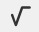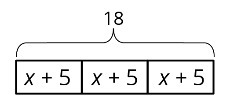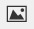PSABM4ZP - Practice---Acc7.3 Lesson 9 Dealing with Negative Numbers (6.EE.A.4, 7.EE.B.4.a)

Solve the equation.

4-28

x=_____

Solve the equation.

x -(-6) = -2

Type your answer below as a number (example: 5, 3.1, 4 1/2, or 3/2):

Solve the equation.Type your answer below as a number (example: 5, 3.1, 4 1/2, or 3/2):

Solve the equation.

-3x + 7 = 1

Type your answer below as a number (example: 5, 3.1, 4 1/2, or 3/2):

Solve the equation.

25+ (-11) -86

Type your answer below as a number (example: 5, 3.1, 4 1/2, or 3/2):
Part A)

Here is an equation 2-15.

Write three different equations that have the same solution as 2-15.

Write the equations using the "WIRIS editor" button.

Part B)

Show or explain how you found them.

Select all the equations that match the diagram.Select all that apply:
Part A)

There are 88 seats in a theater. The seating in the theater is split into 4 identical sections. Each section has 14 red seats and some blue seats.

Draw a tape diagram to represent the situation.

Draw the tape diagram on paper, take a picture, and upload it using the image upload icon.

If you do not have the ability to upload an image of your work type "Diagram is on paper."

Part B)

What unknown amounts can be found by by using the diagram or reasoning about the situation?

Part A)

Match each story to an equation.

1. A stack of nested paper cups is 8 inches tall. The first cup is 4 inches tall and each of the rest of the cups in the stack addsinch to the height of the stack.
2. A baker uses 4 cups of flour. She usescup to flour the counters and the rest to make 8 identical muffins.
3. Elena has an 8-foot piece of ribbon. She cuts off a piece that isof a foot long and cuts the remainder into four pieces of equal length.